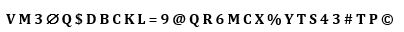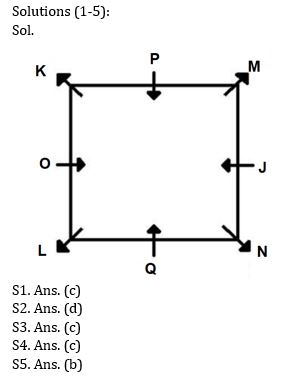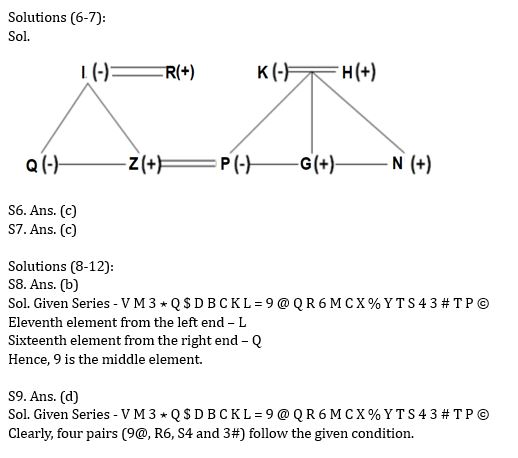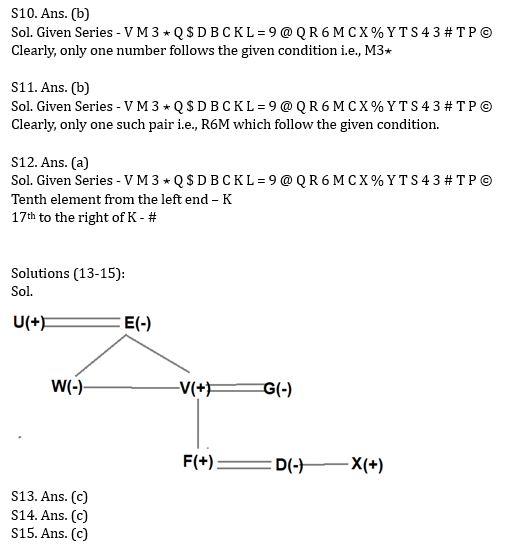Latest Banking jobs   »   Reasoning Quizzes Quiz For IRDA AM...

# Reasoning Quizzes Quiz For IRDA AM 2023- 02rd May

Directions (1-5): Study the information carefully and answer the following questions.
Eight people i.e., J, K, L, M, N, O, P and Q sit around a square-shaped table. Four people sit at the four corners of the table and face outside the table. Four people sit at the middle of the four sides and face inside the table. Only one person sits between J and Q who sits at the middle of the side. K sits third to the left of Q. M sits second to the right of K. Two people sit between M and O. L sits second to the right of N.

Q1. Who sits third to the right of N?
(a) Q
(b) L
(c) O
(d) K
(e) None of these

Q2. Which of the following statements is true?
(a) Q faces M
(b) K sits second to left of J
(c) Two people sit between N and J
(d) O faces J
(e) None is true

Q3. Four of the following five are alike in a certain way and hence form a group. Find the one who does not belong to that group.
(a) Q
(b) P
(c) M
(d) J
(e) O

Q4. How many people sit between P and M when counted from left of P?
(a) Three
(b) One
(c) None
(d) Four
(e) Two

Q5. Who sits second to the left of the one who sits immediate left of J?
(a) N
(b) M
(c) P
(d) K
(e) None of these

Directions (6-7): Study the following information carefully and answer the questions accordingly.
K is the mother of P who is the sister of G. P is married to Z. H is the parent of G. I is the mother of Z and Q is the only daughter of I. N and G are siblings. K has only one daughter. I is the wife of R.

Q6. How is Z related to N?
(a) Son
(b) Sister-in-law
(c) Brother-in-law
(d) Brother
(e) None of these

Q7. How is P related to I?
(a) Sister
(b) Daughter
(c) Daughter-in-law
(d) Mother
(e) Either Sister or Daughter

Directions (8-12): Study the following series carefully and answer the questions given below.Q8. Which of the following element is exactly in the middle of the eleventh element from the left end and sixteenth element from the right end in the above series?
(a) Q
(b) 9
(c) L
(d) P
(e) None of these

Q9. How many such numbers are there in the above series, each of which is either immediately preceded by a letter or immediately followed by a symbol but not both?
(a) One
(b) Two
(c) Three
(d) Four
(e) None of these

Q10. How many such numbers are there in the above series, each of which is immediately preceded by a letter and immediately followed by a symbol?
(a) None
(b) One
(c) Two
(d) Three
(e) None of these

Q11. How many such numbers are there, each of which is immediately preceded and immediately followed by a consonant?
(a) None
(b) One
(c) Two
(d) Three
(e) More than three

Q12. Which of the following element is seventeenth to the right of tenth element from the left end?
(a) #
(b) 3
(c) S
(d) T
(e) None of these

Directions (13-15): Read the following information carefully and answer the questions given below.
A three-generation family consists of eight members i.e., U, V, W, X, D, E, F and G in which three are married couples. V is the father of F. E is the mother of V. W is the sister-in-law of G. G is the mother-in-law of D. F is the grandson of U who is the father-in-law of G. X is the brother-in-law of F.

Q13. What is the relation of W with respect to U?
(a) Daughter-in-law
(b) Niece
(c) Daughter
(d) Granddaughter
(e) None of these

Q14. Who amongst the following is the grandmother of F?
(a) W
(b) G
(c) E
(d) D
(e) None of these

Q15. How many female members in the family?
(a) Five
(b) Three
(c) Four
(d) Can’t be determined
(e) None of the above

Solutions## FAQs

### How many sections are there in the IRDAI exam?

There are 4 sections in the IRDAI assistant manager online preliminary exam. They are Reasoning, English Language, General awareness, Quantitative aptitude.

#### Congratulations!Union Budget 2023-24: Free PDF